# Generate MATLAB Code for Sensitivity Analysis for Design Space Exploration and Evaluation (GUI)

This example shows how to automatically generate a MATLAB function to solve a Sensitivity Analysis evaluation problem. You use the Sensitivity Analyzer to define an evaluation problem for a model of the body's vestibulo-ocular reflex, and generate MATLAB® code to solve this evaluation problem.

### Vestibulo-Ocular Reflex Evaluation Problem

The Identify Key Parameters for Estimation (GUI) example shows how to use the Sensitivity Analyzer to evaluate a cost function for different parameter values in a model of the body's vestibulo-ocular reflex. In this example we load a pre-configured Sensitivity Analyzer session based on that example.

Open the Sensitivity Analyzer for the sdoVOR model:

`ssatool('sdoVOR')`

In the Sensitivity Analyzer, click Open Session and `Open from model workspace`. Open session `sdoVOR_sasessionForEvaluation`.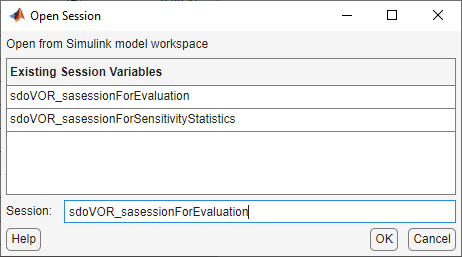This opens a preconfigured session in the Sensitivity Analyzer.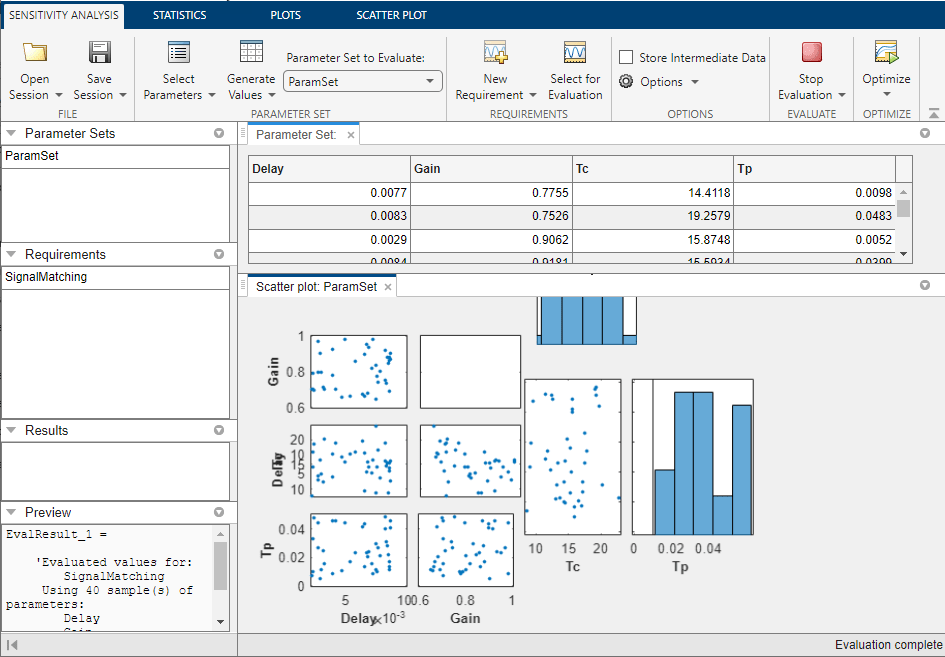### Generate MATLAB Code

From the Evaluate Model list, select `Generate MATLAB Function`.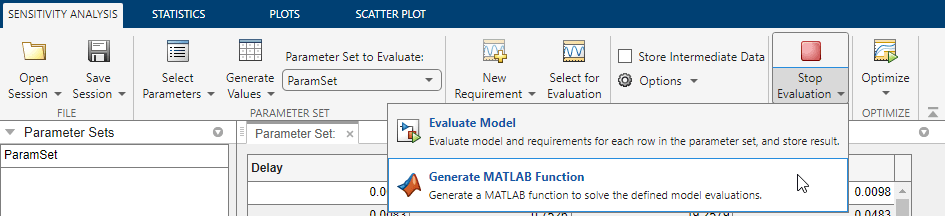The generated code is added to the MATLAB editor as an unsaved MATLAB function.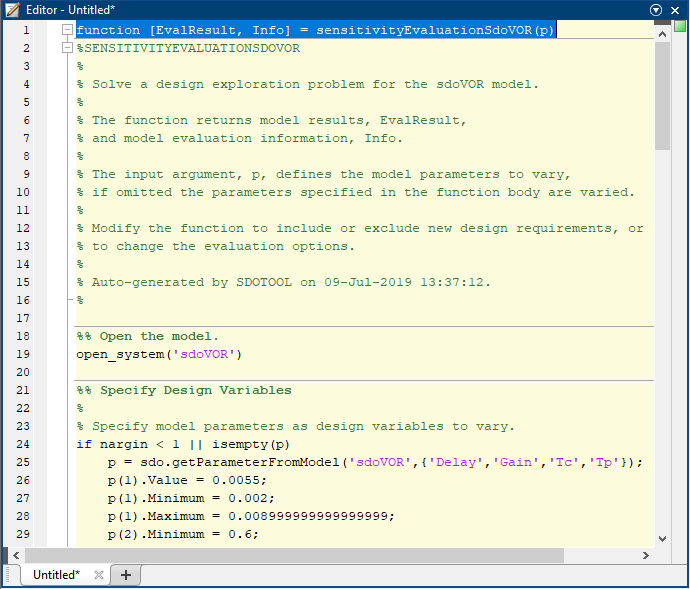Examine the generated code. Significant code portions are:

• Specify Design Variables - Definition of the model parameters being varied.

• Define the Experiments (Signal Matching Requirements) - Definition of the measured and expected signal data to use for signal matching requirements. In this case the signal matching requirement is the only requirement. In other cases, there may be other requirements such as signal bounds.

• Create the Objective Function - Creation of an anonymous function that calls the subfunction `sdoVOR_evalFcn`, which evaluates the model using each experiment and compares simulation and measured experiment outputs. This anonymous function is called by `sdo.evaluate` at each iteration of the evaluation problem to evaluate the model at all combinations of parameters.

• Evaluate the Model - Solve the evaluation problem using the `sdo.evaluate` command.

Select Save from the MATLAB editor to save the generated function.

### Run Generated Code

Run the generated function.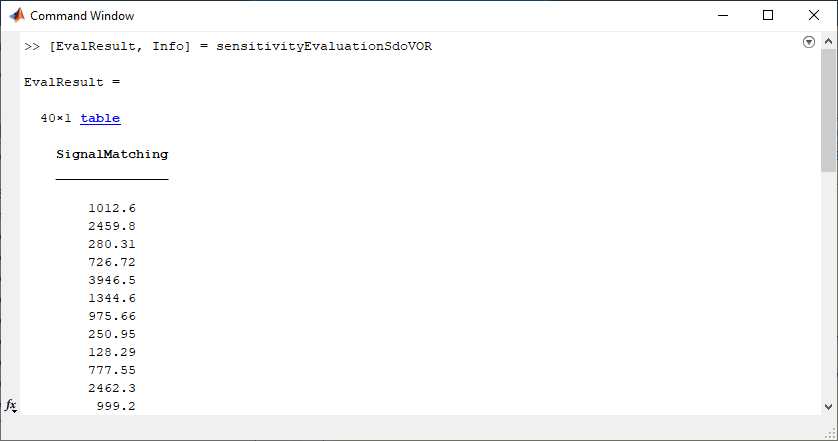The first output argument, `EvalResult`, contains the result of evaluating the model at each combination of parameter values. The second output argument, `Info`, contains information about each evaluation.

### Modify the Generated Code

You can:

• Modify the generated `sensitivityEvaluationSdoVOR` function to include or exclude new experiments or other requirements, or change evaluation options.

• Call the generated `sensitivityEvaluationSdoVOR` function with a different set of parameter values to evaluate.

For details on how to write an objective/constraint function to use with the `sdo.evaluate` command, type `help sdoExampleCostFunction` at the MATLAB command prompt.

Close the model.

## Related Topics